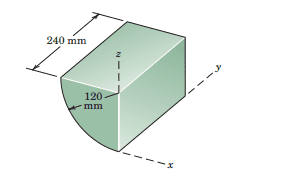# The x,y,z coordinates of CM of a solid cylinderI have found via integration that the y coordinate is $$y =h/2 = 120 mm$$. The x coordinate is $$x = \frac{-4r}{3\pi} = -51.9mm$$ and the z coordinate is $$z = r - \frac{4r}{3\pi} = 69.1 mm$$. I have no answers in my textbook so cant confirm whether i am correct or not.

Last edited:

SteamKing
Staff Emeritus
Homework Helper

Baluncore
If the cylinder is complete then the centre of mass is the midpoint.

If it is a quadrant of a solid cylinder as shown in your picture then it will be at C.y = h/2 as you show. The CM will lie on the plane of symmetry at 45 degrees, so the C.x and C.y offsets from the cylinder axis should have identical values. I suspect your values are correct.
Would it not be easier if you placed your origin and z axis on the cylinder axis ?

The centroid of a cylindrical segment, hoof or wedge is available on Wolfram Alpha. http://mathworld.wolfram.com/CylindricalSegment.html
Unfortunately the quarter tank problem has only one plane cut. You need two.
But one of the values will be the same as for a half cylinder which has a single plane cut.

Baluncore